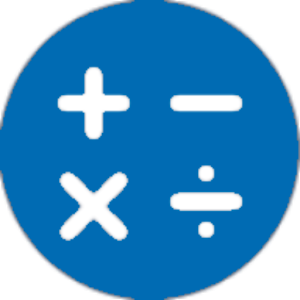Español

Todos los días ofrecemos apliaciones y juegos licenciados GRATIS para Android de otra manera tendrás que comprarlo.\$2.49
EXPIRÓ

# Android Giveaway of the Day - NT Calculator - Extensive Calculator Pro

Easily handle all the calculations necessary for everyday life with a single application
\$2.49 EXPIRÓ
Votación de Usuario: 6 (67%) 3 (33%)

Esta oferta de regalo ha expirado. NT Calculator - Extensive Calculator Pro ya está disponible.

Dear you,

This Extensive Calculator app allows you to easily handle all the calculations necessary for everyday life with a single application. A Calculator app with a simple interface and practical functions! It can replace your default calculator.

With Extensive Calculator Pro on your Android, you do not need to bring your calculator anymore. This calculator app serves you everything from A - Z.

List of calculators currently supported:
1. Standard Calculator
• Quick and easy.
• History available.
• Simple interface
• Basic features such as: %, +, -, x, /, 0 - 9, ( and ).
Ex: -(2+4)x4 = -24

2. Scientific Calculator
• Basic arithmetic operations including percentage, modulo and negation;
• History available.
• Supports the four fundamental arithmetic operations, square, formula's parenthesis, and simple scientific operations such as trigonometric and logarithmic functions.
• All features such as: sin, cos, tan, %, !, 0-9, (, ), RAD and DEG, log, ln, Mod, +, -, x, /, SQRT, SQR, signed,...
• In scientific calculator, It has a button to change RAD to DEG and contrary.
• You can use EXP is E (sam as 10 ^ ) to calculate large decimal number. If you need to get previous result of calculator, you can use ANS button.

3. Loan Calculator
• Simple interface
• Suitable for car, bank loan
• Three primary input is loan amount, interest rate, loan term
• Loan calculator show you the amount paid per month and total interest.

4. Converter
Supports many Unit of measurement commonly used in everyday life such as:
• weight converter: kg to st, lbs to grams, lbs to kilograms, stones to grams,...
• length converter: size converter, meters to kilometers, mile in km, km in m, inch in cm, ...
• speed converter: km/h, cm/s, m/s, m/s to km/h, ft/s, ft to m, ...
• temperature converter: c to f, f to c, c to kelvin, ...
• data converter: byte to bit, bit to byte, ...
• area converter: km2 to m2, 1 hectare to km2, cm2 to m2, mm2 to m2, ...
• time converter: minute to second, year to second, millisecond to second, ...
• energy converter: joule, kwh, ...
• pressure converter: atmospheres, bars, kilopascals, pascals, millimeters of mercury, pound per square inch
• volume converter: gallons to qts, teaspoon, cups, liters, cubic inches, cubic yards, cubic feet, quarts, pints, fluid ounces (US and UK),...

Why doesn't Extensive Calculator use flat design ? Because it make the numbers hard to see on your screen.

Thank for using Extensive Calculator app, if you have questions I ready to answer you anytime. I hope you feel happy and useful when using this calculator app.

The list will be increased!

Thanh Le Tran Ngoc

Productivity

2.3

### Tamaño:

2.7M

Everyone